# Discount and Tax Calculator – This app can calculate discount price with tax easily.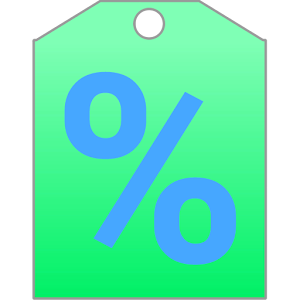This app can calculate discount price with tax easily.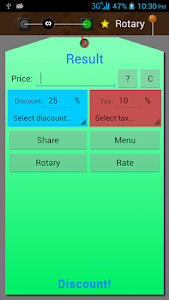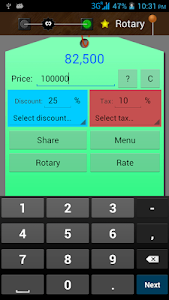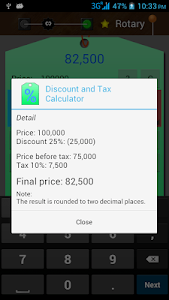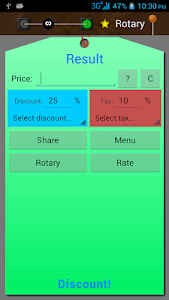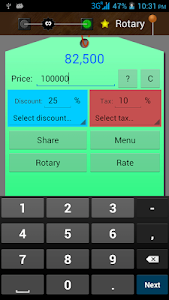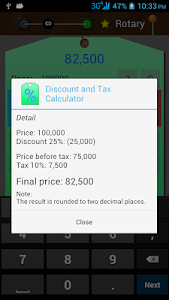This app can calculate discount price with tax easily. First, discount price is calculated then added with tax.

Example:
Item with price 100000 which have 25% discount with 10% tax will be calculated as 82500.
100000 with 25% discount = 75000, then 75000 with 10% tax = 82500

Note:
* The result is rounded to two decimal places.